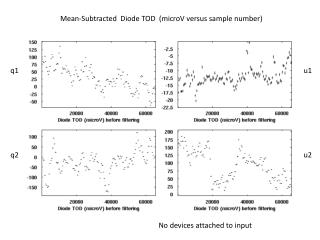DownloadDownload PresentationMean-Subtracted Diode TOD ( microV versus sample number)

# Mean-Subtracted Diode TOD ( microV versus sample number)

Download Presentation## Mean-Subtracted Diode TOD ( microV versus sample number)

- - - - - - - - - - - - - - - - - - - - - - - - - - - E N D - - - - - - - - - - - - - - - - - - - - - - - - - - -
##### Presentation Transcript

1. Mean-Subtracted Diode TOD (microV versus sample number) q1 u1 q2 u2 No devices attached to input

2. Mean-Subtracted Diode RMS (microV) q1 u1 q2 u2

3. No Devices Connected to Input filter out f < 5 Hz

4. Mean-Subtracted Diode TOD After Filtering f < 5 hz (microV versus sample number)

5. Mean-Subtracted Diode RMS After Filtering (microV) 10 microvolt RMS

6. Analog Devices AD8666 Noise Calculation Rated Noise = 8 –60 nV/sqrt(Hz) for 10 < f<10 KHz. Bandwidth of circuit = 20 KHz sqrt(BW) = 141 /sqrt(sec) Expected noise = 1-8 microvolt RMS per preamp 2 preamps implies additional sqrt(2) Expected noise = 1.4 – 12 microvolt Measured noise for f >5Hz =10 microvolt RMS